How to Learn in 24 Hours?The Rapid Learning Movie

 Need Help? M-F: 9am-5pm(PST): Toll-Free: (877) RAPID-10 US Direct: (714) 692-2900 Int'l: 001-714-692-2900 24/7 Online Technical Support: The Rapid Support Center Secure Online Order:Need Proof? Testimonials by Our Users

 Rapid Learning Courses: MCAT in 24 Hours (2015-16) USMLE in 24 Hours (Boards) Chemistry in 24 Hours Biology in 24 Hours Physics in 24 Hours Mathematics in 24 Hours Psychology in 24 Hours SAT in 24 Hours ACT in 24 Hours AP in 24 Hours CLEP in 24 Hours DAT in 24 Hours (Dental) OAT in 24 Hours (Optometry) PCAT in 24 Hours (Pharmacy) Nursing Entrance Exams Certification in 24 Hours eBook - Survival Kits Audiobooks (MP3)

 Tell-A-Friend: Have friends taking science and math courses too? Tell them about our rapid learning system.Home »  Mathematics »  College Algebra

Polynomial Functions and Factoring

 Topic Review on "Title": Monomial (in one variable X): A function of the form:,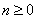. Polynomial: Any finite sum of monomials of the form:Coefficients: The (real or complex) numbers of the form: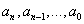Constant term: The zero coefficient is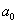. The product of polynomials: The product is defined according to the rules: 1)2)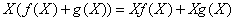. Degree: The degree n is such that:Dividing polynomials: We say that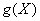dividesif there is a polynomial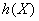such that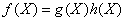. Roots: Real or complex numbers where polynomials equal to 0. Factorization of roots: If a polynomial of degree n has roots: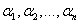then it can be factored as: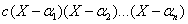. Fundamental theorem of algebra: A polynomial of degree n has exactly n complex roots. Rolle’s Theorem: Let f(X) be a polynomial and a, b are two numbers such thatand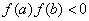then there is a number c such that :Rapid Study Kit for "Title":
 Flash Movie Flash Game Flash Card Core Concept Tutorial Problem Solving Drill Review Cheat Sheet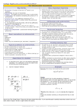"Title" Tutorial Summary : This tutorial shows the basic definitions of sum and product of polynomials along with their degrees. Through the use of examples the important aspects of polynomials are presented. The division and factoring of polynomials is shown by techniques shown in the examples. The principles of computing polynomial quotients are seen in this tutorial. The roots of polynomials are defined with the use of examples. Important theorems such as Rolle’s Theorem are discussed to emphasize the importance of the polynomial roots.

 Tutorial Features: Specific Tutorial Features: • Problem-solving techniques are used to work out and illustrate the example problems, step by step. • Easy explanation for sometimes confusing mathematics formulas. • Example showing the division algorithm. Series Features: • Concept map showing inter-connections of new concepts in this tutorial and those previously introduced. • Definition slides introduce terms as they are needed. • Visual representation of concepts • Animated examples—worked out step by step • A concise summary is given at the conclusion of the tutorial.

 "Title" Topic List: Basic definitions of polynomials The degree of polynomials Division and factoring Dividing polynomials Methods to divide polynomials Roots Factorization of roots Complex roots Rolle’s Theorem

See all 24 lessons in College Algebra, including concept tutorials, problem drills and cheat sheets:
Teach Yourself College Algebra Visually in 24 Hours